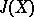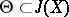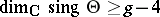Poincaré divisor

The divisor given by the natural polarization over the Jacobian (cf. Jacobi variety) of an algebraic curve. The intersection form of one-dimensional cycles in the homology of an algebraic curveinduces a unimodular skew-symmetric form on the lattice of periods. By the definition of a polarized Abelian variety (cf. Polarized algebraic variety) this form determines the principal polarization over the Jacobianof the curve. Therefore the effective divisorgiven by this polarization is uniquely determined up to translation by an element. The geometry of the Poincaré divisorreflects the geometry of the algebraic curve. In particular, the set of singular points of the Poincaré divisor has dimension, whereis the genus of the curve(see ).